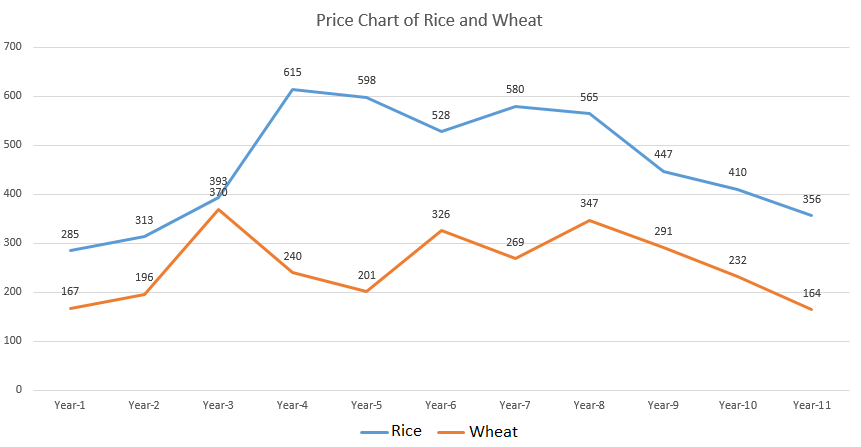# DI LR Questions for CAT

0
6592

DI LR Questions for CAT:

Logical Reasoning is the second section in CAT exam. Around 16 questions will be asked from this topic in the form of 3-5 sets having 3-5 questions in each LR set. This section tests the logical ability of a candidate to solve a given problem. LR (Logical Reasoning) questions can be considered as puzzles. They mainly have questions from the following topics.

Instructions:

Given in the below chart are prices(in Rupee/100kg) of rice and wheat over a period of 11 years. Answer the following questions based on the given information.Question 1:

If the price of rice in year-1 is greater than that of wheat in year-1 by X% and the price of rice in year-11 is greater than that of wheat in year-11 by Y%, then by how many percentage points is X lesser than Y?

a) 13.54
b) 29.39
c) 23.1
d) None of these

Question 2:

If the greatest percentage difference between the price of rice and wheat(w.r.t wheat) in any year is X and the smallest percentage difference between the price of rice and wheat(w.r.t to wheat) in any year is Y, then by what percentage(closest integer) is Y less than X?

a) 94%
b) 95%
c) 96%
d) 97%

Question 3:

If M is the number of years that both the curves showed a similar trend in the prices and N is the number of years that both the curves showed different trend in the prices, then find the value of |M – N|.

a) 0
b) 2
c) 4
d) 6

Question 4:

Raghu buys 100kg of rice in year 1 and sells them in year 2 at the price in year 2. He buys 100 kg of wheat in year 2 and sells them in year 3 at the price in year 3. He buys 100 kg of rice in year 3 and sells them in year 4 at the price in year 4. This process is continued until he sells 100 kg of wheat in year-11. Find the total profit percentage he gets.

a) 1.69%
b) 1.96%
c) 2.25%
d) 2.56%

Solutions 1 to 4

X = $$\frac{118}{167}*100$$ = 70.6%
Y = $$\frac{192}{164}*100$$ = 117%
Percentage points difference => Y – X = 117 – 70.6 = 46.4

We can see that the greatest price difference is in year 5 => Percentage difference = $$\frac{598-201}{201}*100$$ = 197.5%
Least percentage difference is in year-3 => percentage difference = $$\frac{23}{370}*100$$ = 6.2%
The difference = 197.5 – 6.2 = 191.3
% difference = $$\frac{191.3}{197.5}*100$$ = 97%

We can see that the prices of both the commodities either increase or decrease in 6 years => M = 6
The price of one of the commodity increases and the price of the other decreases in 4 years => N = 4
Hence, |M – N| = 2

Total cost price of Rice = 285 + 393 + 598 + 580 + 447 = Rs 2303
Total selling price of Rice = 313 + 615 + 528 + 565 + 410 = Rs 2431
Total cost price of Wheat = 196 + 240 + 326 + 347 + 232 = Rs 1341
Total selling price of Wheat = 370 + 201 + 269 + 291 + 164 = Rs 1295
Total cost price = 2303 + 1341 = 3644
Total selling price = 2431 + 1295 = Rs 3726
Total Profit = 3726 – 3644 = Rs 82
Profit % = $$\frac{82}{3644}*100$$ = 2.25%

Instruction (5 to 6)

Answer the questions based on the following information:

Five famous paintings – Mona Lisa, The Scream, The Starry Night, Guernica and The Last Supper by five famous artists – Leonardo, Michael, Ravi, Pablo and Hussain – were being auctioned. Five people – Ram, Shyam, Bheem, Mohan and Krishna – bought one painting each. The following information is known:

1. Krishna bought Mona Lisa, but it was painted by neither Ravi nor Pablo
2. Ram did not buy The Starry Night but he bought the painting by Leonardo
3. Hussain painted The Scream but it was not bought by Shyam
4. Mohan did not buy the Scream and also not the painting by Pablo

Question 5:

If Mohan bought Guernica, then who bought the painting the Starry Night?

a) Bheem
b) Ram
c) Shyam
d) None of the above

Question 6:

Which of the following paintings did Shyam buy?

a) The painting by Pablo
b) The painting by Hussain
c) The Starry Night
d) Cannot be determined

Solutions 5 to 6

From the information given above, the paintings, their artists and the persons who bought them are as follows:

Krishna – Mona Lisa – Michael
Ram – The Last Supper – Leonardo
Bheem – The Scream – Hussain
Mohan – Guernica – Ravi
Shyam – The Starry Night – Pablo
So, Shyam bought The Starry Night

From the information given above, the paintings, their artists and the persons who bought them are as follows:
Krishna – Mona Lisa – Michael
Ram – Guernica / The Last Supper – Leonardo
Bheem – The Scream – Hussain
Mohan – The Starry Night / Guernica / The Last Supper – Ravi
Shyam – The Starry Night / Guernica / The Last Supper – Pablo
So, Shyam bought the painting by Pablo